# Hidden Markov Models Training – The Forward-Backward Algorithm

## Learning

Given an observation sequence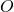and the set of possible states in the HMM, learn the HMM parameters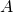and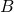.

The standard algorithm for HMM training is the Forward-Backward, or Baum-Welch algorithm. It is an iterative algorithm, computing an initial estimate for the probabilities, then using those estimates to computing a better estimate, and so on, iteratively improving the probabilities that it learns.

## Backward Probability

The backward probability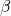is the probability of seeing the observations from time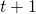to the end, given that we are in stateat time(and given the HMM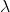):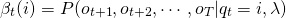The state at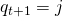can be any of N states.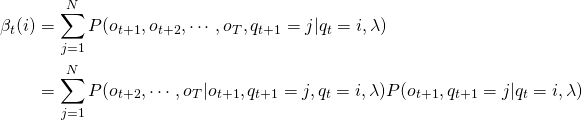Observations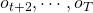are independent of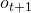and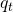.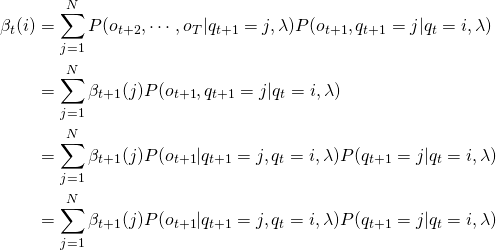Observationis independent of.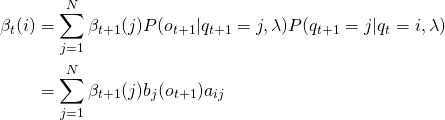## Visualization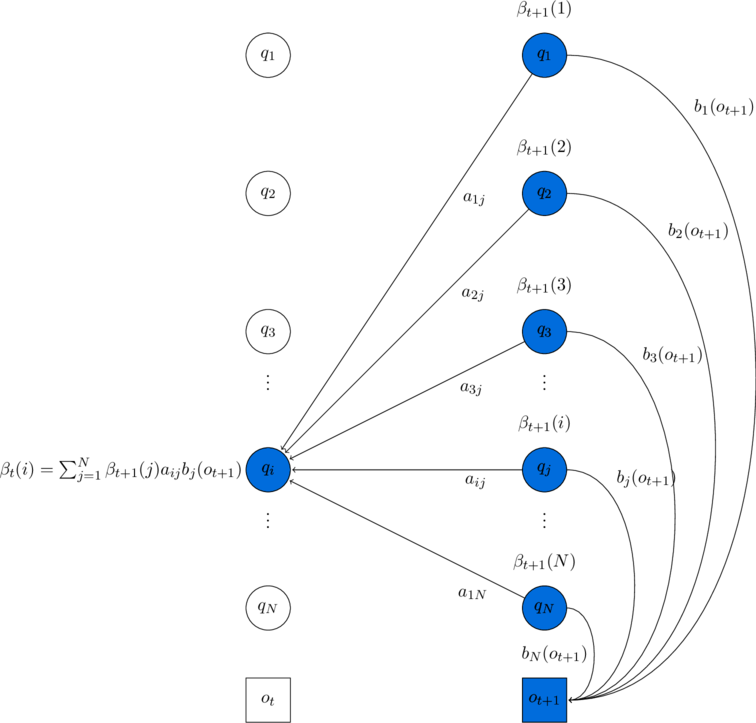The computation of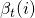by summing all the successive values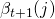weighted by their transition probabilities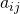and their observation probabilities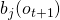. Hidden states are in circles, observations in squares. Shaded nodes are included in the probability computation for.

## Initialization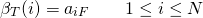## Recursion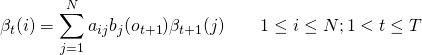## Termination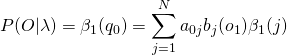## Probability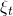Define the probabilityas the probability of being in stateat timeand state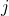at time, given the observation sequence and the model.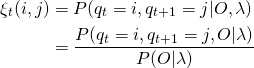The observationis defined as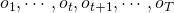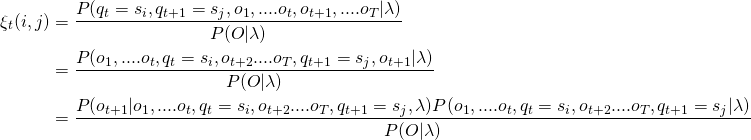The observation, emitted at state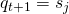, will only depend on the state. Simplifying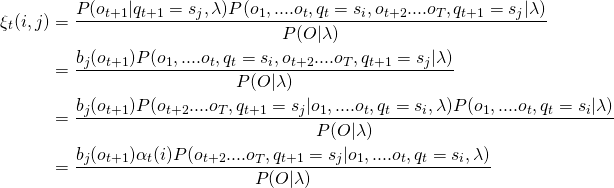The observations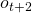to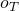and the statedo not depend on any observation from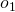to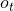. Simplifying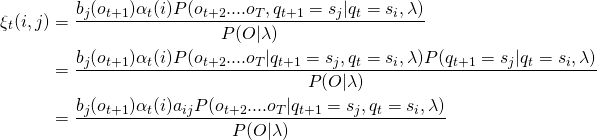The observationstodo not depend on the state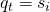. Simplifying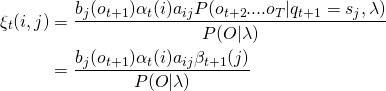## Visualization

\clearpage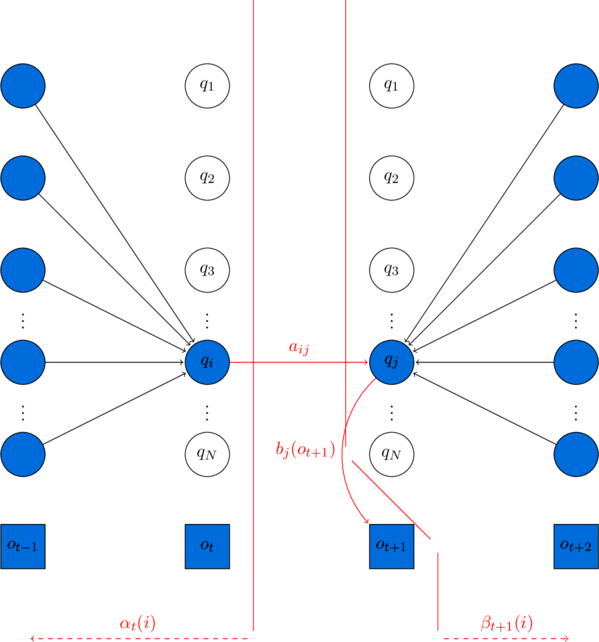Computation of the joint probability of being in stateat timeand stateat time. The figure shows the various probabilities that need to be combined to produce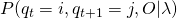: the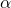andprobabilities, the transition probability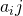and the observation probability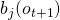. Hidden states are in circles, observations in squares. Shaded nodes are included in the probability computation for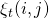.

The probability of the observation given the model is simply the forward probability of the whole utterance (or alternatively, the backward probability of the whole utterance):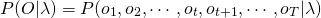The state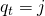can be any of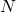possible states.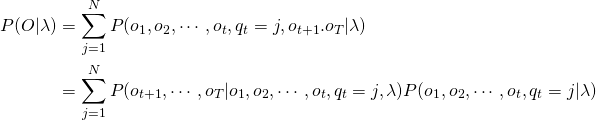Observations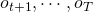do not depend on observations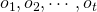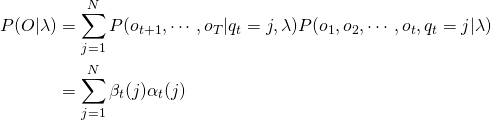Define the probability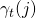as the probability of being in stateat time.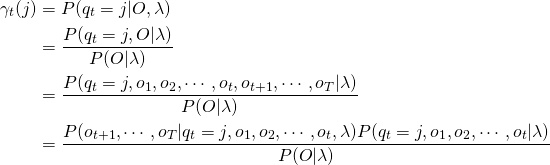Observationsdo not depend on observations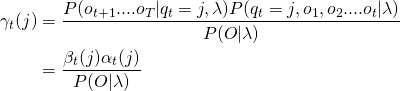## Estimate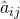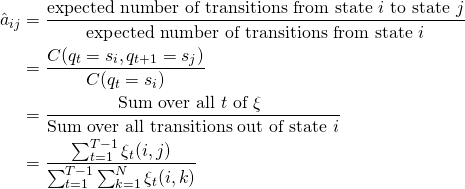## Estimate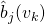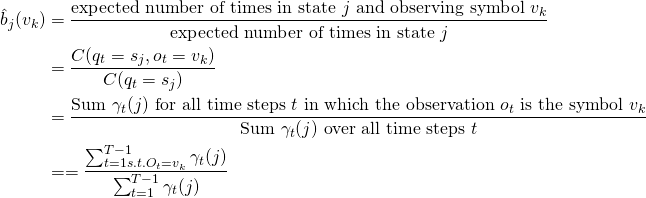The forward-backward algorithm has two steps: the expectation step, or E-step, and the maximization step, or M-step.

In the E-step, the expected state occupancy count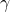and the expected state transition count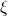from the earlierandprobabilities are computed.

In the M-step,andare used to recompute newandprobabilities.